# 17 a 37 kg block on a horizontal frictionless surface

• Test Prep
• 21
• 87% (23) 20 out of 23 people found this document helpful

This preview shows page 3 - 5 out of 21 pages.

17)A 3.7 kg block on a horizontal frictionless surface is attached to a spring whose force constant is450 N/m. The block is pulled from its equilibrium position at x=0 m to a displacementx= +0.080 m and is released from rest. The block then executes simple harmonic motion along thex-axis (horizontal). The maximum elastic potential energy of the system is closest to:17)A)1.6 JB)1.8 JC)1.3 JD)1.7 JE)1.4 J
18)If a wave has a wavelength of 25.4 cm and a frequency of 1.63 kHz, its speed is closest to18)
19)If a wave has a speed of 362 m/s and a period of 4.17 ms, its wavelength is closest to19)
20)The speed of sound in steel is 5000 m/s. What is the wavelength of a sound wave of frequency660 Hz in steel?20)
21)Crests of an ocean wave pass a pier every 10.0 s. If the waves are moving at 5.6 m/s, what is thewavelength of the ocean waves?21)A)28 mB)56 mC)48 mD)64 m
22)Transverse waves propagate at 43.2 m/s in a string that is subjected to a tension of 60.5 N. If thestring is 15.9 m long, what is its mass?22)
23)Which of the following is a FALSE statement?23)
3
##### We have textbook solutions for you!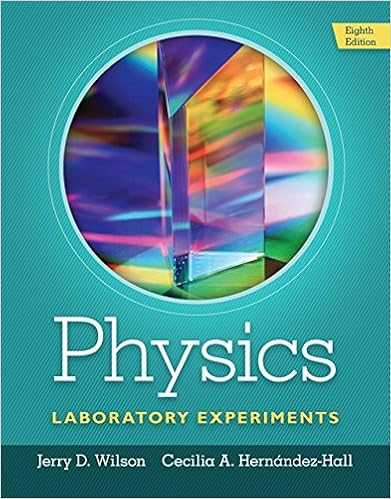The document you are viewing contains questions related to this textbook.
Chapter 14 / Exercise 01
Physics Laboratory Experiments
WilsonExpert Verified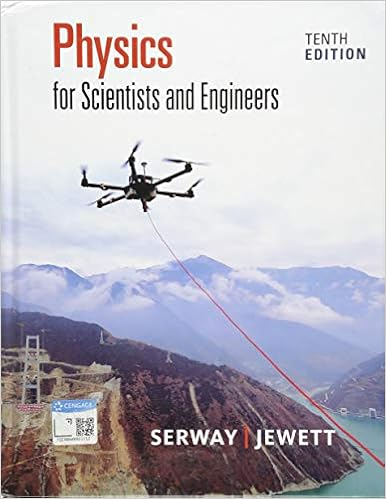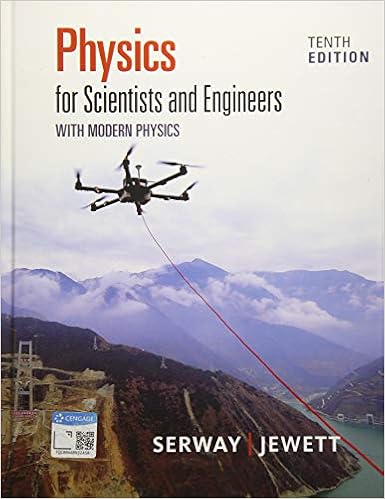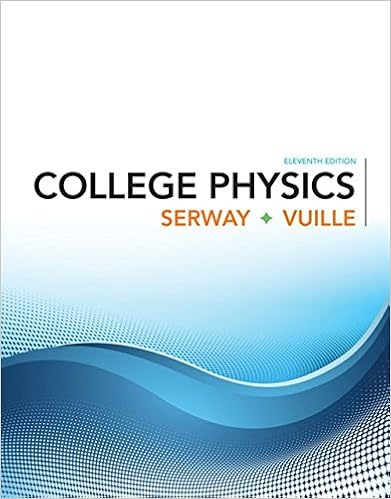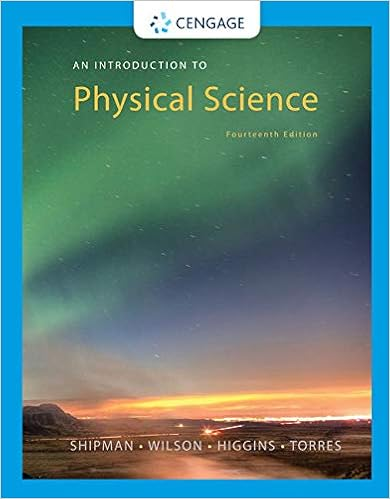Browse all Textbook Solutions
Use Figure 2.1 to answer the following question(s).Figure 2.124)The graph in Figure 2.1 shows the position of an object as a function of time. The letters H-Lrepresent particular moments of time.At which moment in time is the speed of the object thehighest?24)
25)The graph in Figure 2.1 shows the position of an object as a function of time. The letters H-Lrepresent particular moments of time. At which moment in time is the speed of the object equal tozero?25)A)LB)IC)HD)KE)J
26)Which of the following situations is impossible?26)
27)A racing car accelerates uniformly from rest along a straight track.This track has markers spacedat equal distances along it from the start, as shown in Figure 2.2.The car reaches a speed of 140km/h as it passes marker 2.Figure 2.2Whereabouts on the track was the car when it was travelling at half this speed, i.e. at 70 km/h?

Course Hero member to access this document

Course Hero member to access this document

End of preview. Want to read all 21 pages?

Course Hero member to access this document

Term
Spring
Professor
CRISS
##### We have textbook solutions for you!
The document you are viewing contains questions related to this textbook.The document you are viewing contains questions related to this textbook.
Chapter 14 / Exercise 01
Physics Laboratory Experiments
WilsonExpert Verified
•••